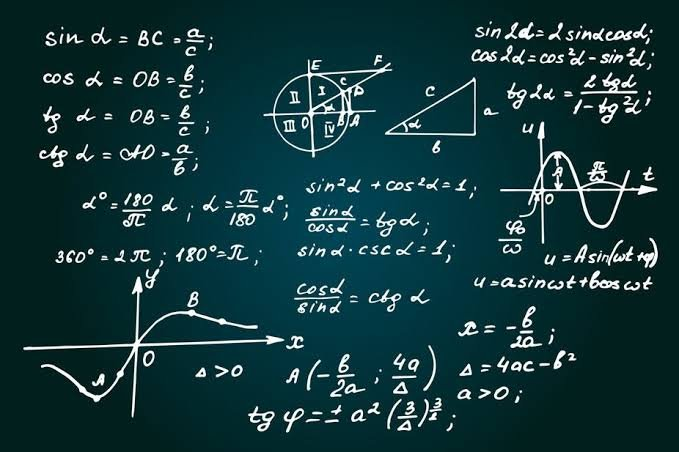# Mathematicians changed the world with unprecedented theories

in #actnearn2 years ago

Mathematics is known by some scientists as the science of measurement, arithmetic and geometry is a science that indicates the quantum and number, is the study of numbers and patterns and also called the language of the universe, mathematics has a great place because it is used in all areas of scientific, social and natural life, many mathematicians contributed to its development Throughout history, mathematics is the foundation of almost all science

Do you know the most important mathematicians?
Pythagoras

Pythagoras is the 25 most famous mathematician in the world.He is also known as the father of modern mathematics. Engineering.

Leonardo Pisano

He is an Italian mathematician nicknamed the Modern World because he introduced the Arabic numbering system and published it in Europe, famous for the Fionache sequence and has many contributions to the development of modern mathematics.

Isaac Newton
He is one of the most famous mathematicians and physicists in history.Image Source

Sort:

You got a 20.30% upvote from @minnowvotes courtesy of @mr-writer!

This post earned a total payout of 0.032\$ and 0.024\$ worth of author reward which was liquified using @likwid. To learn more.